# RD Sharma Solutions for Class 11 Chapter 23 - The Straight Lines Exercise 23.3

In this section, we shall learn about one of the different forms of the equation of a straight line i.e., slope intercept form of a line with illustrations, for better understanding of the concepts. The solutions here are formulated by the experts to help students solve textbook problems without any difficulty. Students who are not able to clear doubts during class hours can clear them by referring to the solutions. Students can effortlessly download the RD Sharma Class 11 Maths Solutions pdf from the links provided below and start practising offline, to yield good results in the board exams.

## Download the pdf of RD Sharma Solutions for Class 11 Maths Exercise 23.3 Chapter 23 – The Straight Lines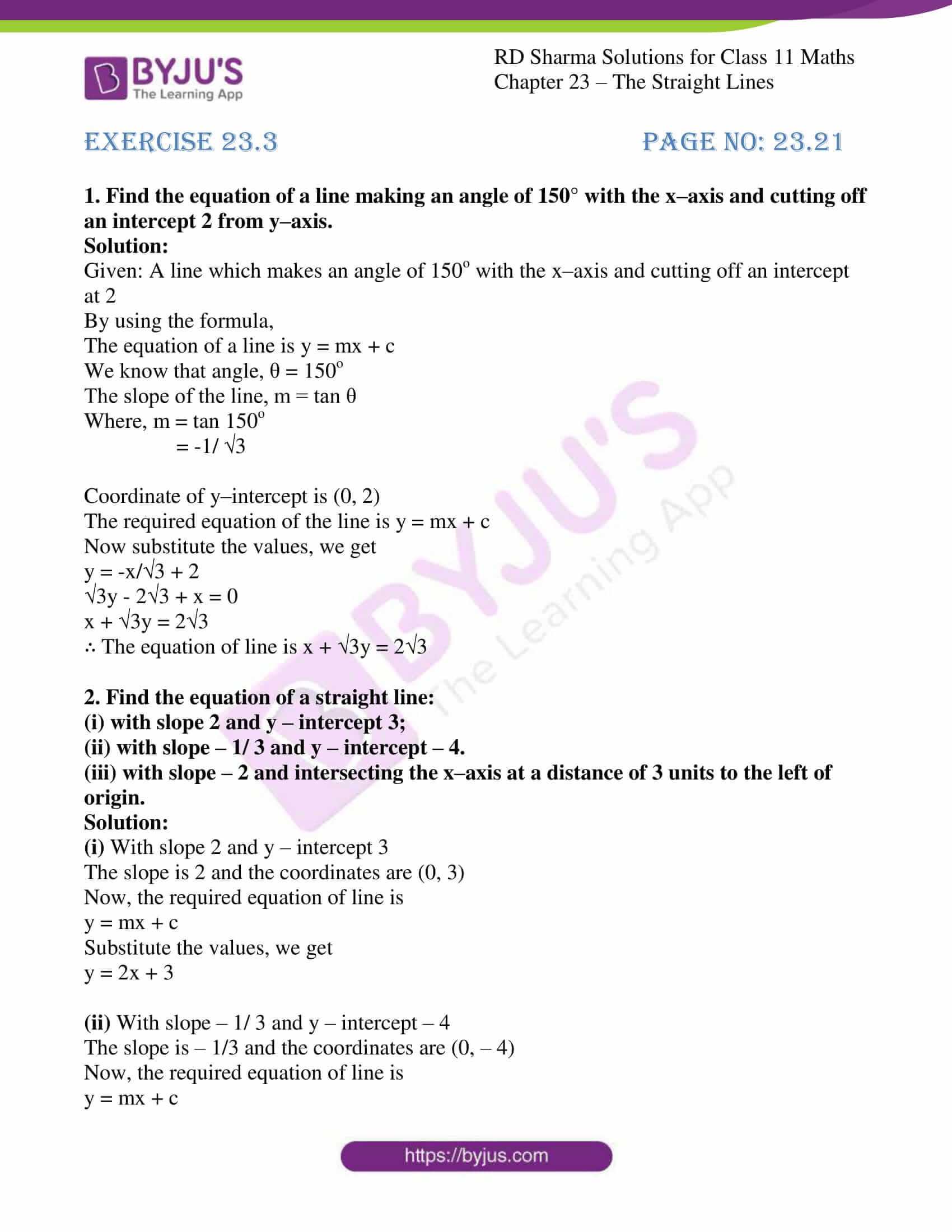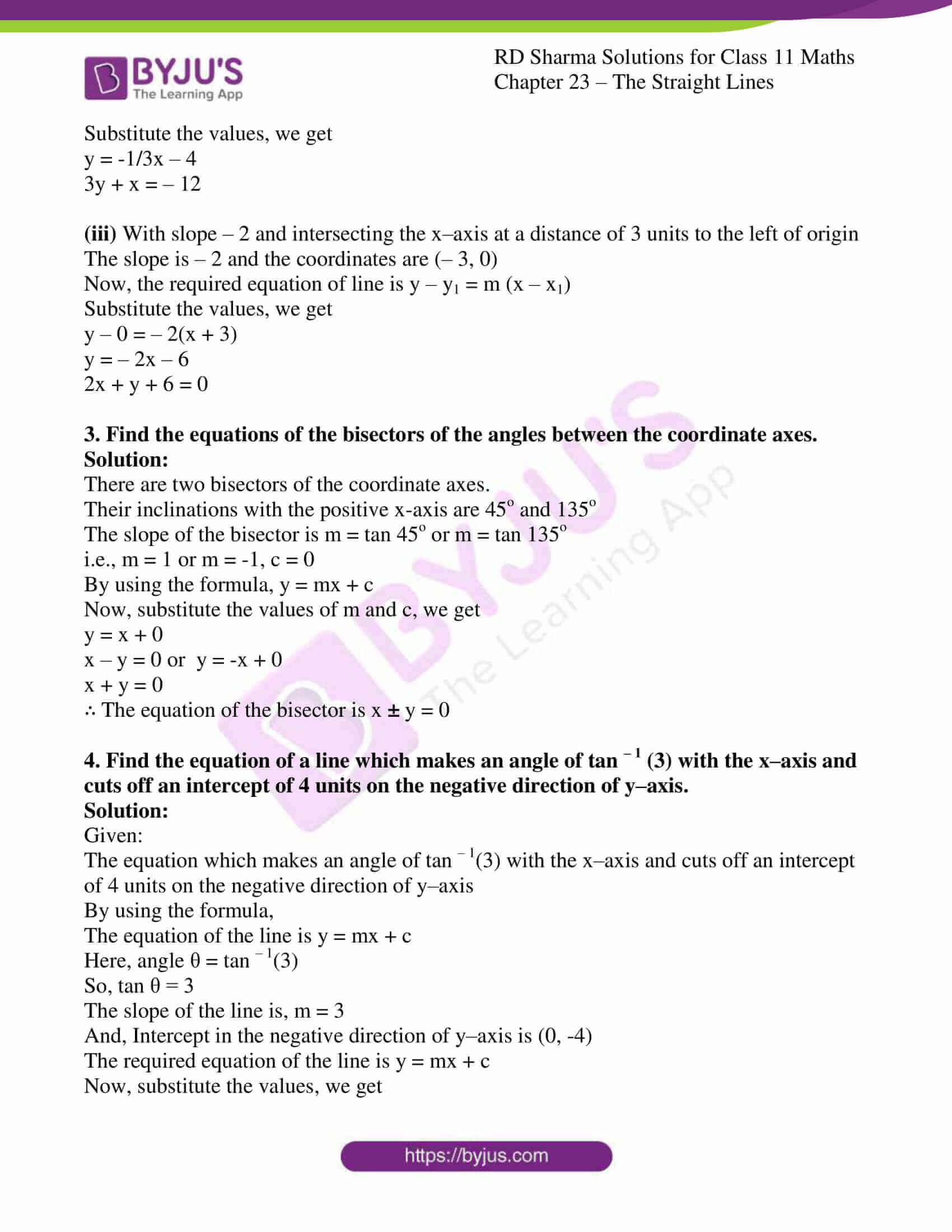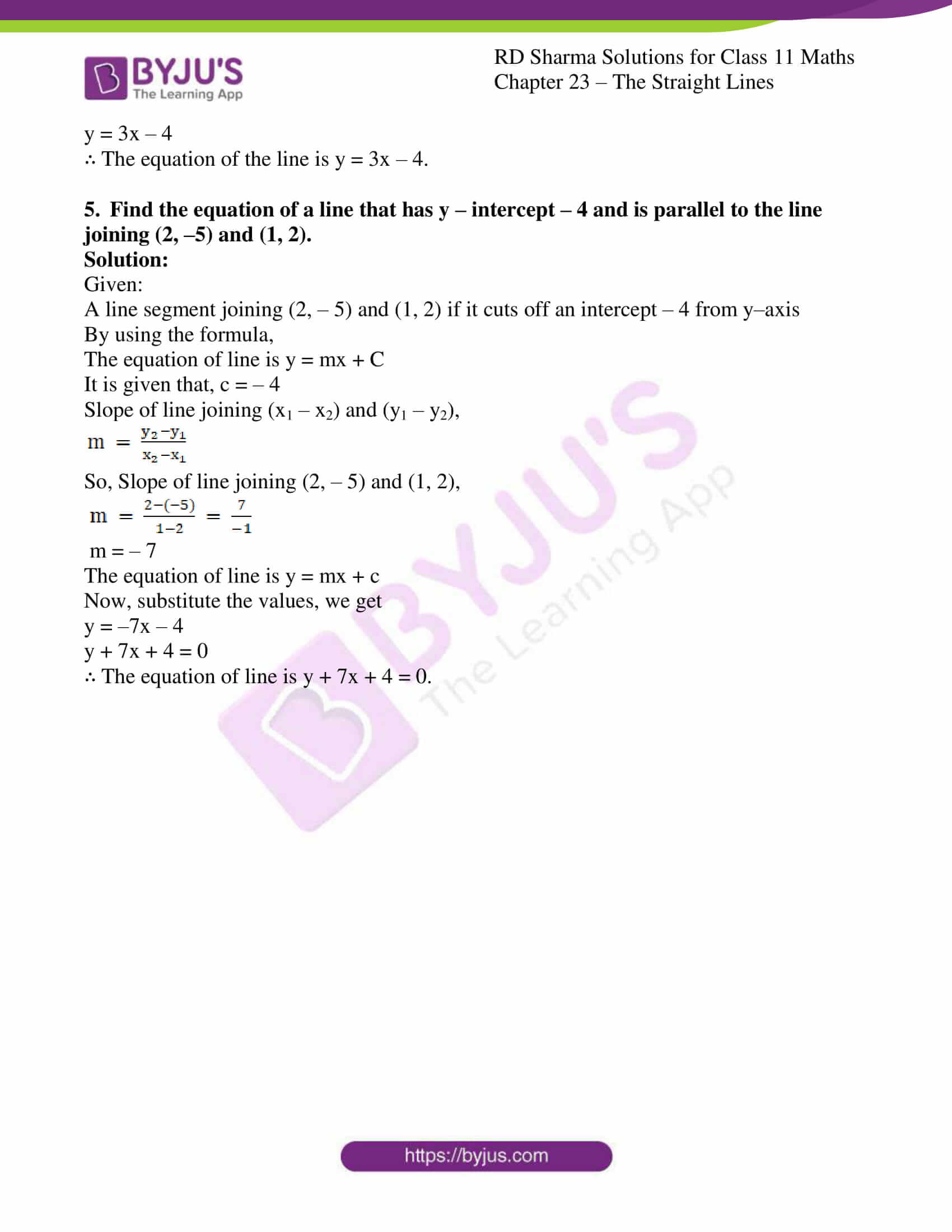### Access answers to RD Sharma Solutions for Class 11 Maths Exercise 23.3 Chapter 23 – The Straight Lines

#### EXERCISE 23.3 PAGE NO: 23.21

1. Find the equation of a line making an angle of 150° with the x–axis and cutting off an intercept 2 from y–axis.

Solution:

Given: A line which makes an angle of 150o with the x–axis and cutting off an intercept at 2

By using the formula,

The equation of a line is y = mx + c

We know that angle, θ = 150o

The slope of the line, m = tan θ

Where, m = tan 150o

= -1/3

Coordinate of y–intercept is (0, 2)

The required equation of the line is y = mx + c

Now substitute the values, we get

y = -x/3 + 2

3y – 23 + x = 0

x + 3y = 23

∴ The equation of line is x + 3y = 23

2. Find the equation of a straight line:
(i) with slope 2 and y – intercept 3;
(ii) with slope – 1/ 3 and y – intercept – 4.
(iii) with slope – 2 and intersecting the x–axis at a distance of 3 units to the left of origin.

Solution:

(i) With slope 2 and y – intercept 3

The slope is 2 and the coordinates are (0, 3)

Now, the required equation of line is

y = mx + c

Substitute the values, we get

y = 2x + 3

(ii) With slope – 1/ 3 and y – intercept – 4

The slope is – 1/3 and the coordinates are (0, – 4)

Now, the required equation of line is

y = mx + c

Substitute the values, we get

y = -1/3x – 4

3y + x = – 12

(iii) With slope – 2 and intersecting the x–axis at a distance of 3 units to the left of origin

The slope is – 2 and the coordinates are (– 3, 0)

Now, the required equation of line is y – y1 = m (x – x1)

Substitute the values, we get

y – 0 = – 2(x + 3)

y = – 2x – 6

2x + y + 6 = 0

3. Find the equations of the bisectors of the angles between the coordinate axes.

Solution:

There are two bisectors of the coordinate axes.

Their inclinations with the positive x-axis are 45o and 135o

The slope of the bisector is m = tan 45o or m = tan 135o

i.e., m = 1 or m = -1, c = 0

By using the formula, y = mx + c

Now, substitute the values of m and c, we get

y = x + 0

x – y = 0 or y = -x + 0

x + y = 0

∴ The equation of the bisector is x ± y = 0

4. Find the equation of a line which makes an angle of tan – 1 (3) with the x–axis and cuts off an intercept of 4 units on the negative direction of y–axis.

Solution:

Given:

The equation which makes an angle of tan – 1(3) with the x–axis and cuts off an intercept of 4 units on the negative direction of y–axis

By using the formula,

The equation of the line is y = mx + c

Here, angle θ = tan – 1(3)

So, tan θ = 3

The slope of the line is, m = 3

And, Intercept in the negative direction of y–axis is (0, -4)

The required equation of the line is y = mx + c

Now, substitute the values, we get

y = 3x – 4

∴ The equation of the line is y = 3x – 4.

5. Find the equation of a line that has y – intercept – 4 and is parallel to the line joining (2, –5) and (1, 2).

Solution:

Given:

A line segment joining (2, – 5) and (1, 2) if it cuts off an intercept – 4 from y–axis

By using the formula,

The equation of line is y = mx + C

It is given that, c = – 4

Slope of line joining (x1 – x2) and (y1 – y2),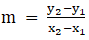So, Slope of line joining (2, – 5) and (1, 2),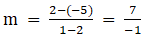m = – 7

The equation of line is y = mx + c

Now, substitute the values, we get

y = –7x – 4

y + 7x + 4 = 0

∴ The equation of line is y + 7x + 4 = 0.